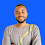# MATH QUIZ: The tricky viral Math Quiz 3 - 3 × 0 + 3 ÷3 =? Solved

3
Hi friends, today i will show you how to solve this type of tricky Math Quiz. The Quiz looks simple but very tricky. To solve this type we only need to apply BODMAS OR PEMDAS rules.

Here is ths Quiz for today.
3 - 3 × 0 + 3 ÷ 3 = ?.

This particular Quiz has gone viral when it was first published online and so many people failed. Many people had 1 and some 0 but read this article carefully and follow the solving steps below to know why the correct answer is 4.
Now let's get solving with the use of BODMAS.

3-3×0+3÷3 =? ( BODMAS)
Step I (division)
3÷3=1
Rearrangement
3-3×0+1
Step II ( multiplication)
3×0=0
Rearrangement
3-0+1
Rearrangement
3+1-0
3+1=4
Rearrangement
4-0
Step IV (Subtraction)
4-0
Answer = 4. If you still have a different view please comment.
Tags

1.2.1.